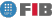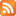# Calculus (CAL)

Credits Dept. Type Requirements
9.0 (7.2 ECTS) MAT
• Compulsory for DIE
• Compulsory for DCSFW
• Compulsory for DCSYS

## Instructors

 Person in charge: (-) Others: (-)

## General goals

It is quite possible that computer engineers will encounter problems that involve making calculations at some point in their professional careers. Despite the fact that IT experts do not have to make these sorts of calculations-but rather have them done for them-they still need to be able to implement them. For this reason it is useful for them to understand these calculations and to know where to look for relevant information. In some subjects in this course, students have to know how to perform certain calculations without starting from square one. Thus, the overall goal of the subject is that students in information technology come away with an understanding and ability to work with the fundamental concepts and techniques of mathematics. In particular, the subject aims for students to understand and be able to use the concept of functions with one or more variables.

## Specific goals

### Knowledges

1. Learn the properties and graphic representation of elementary functions.
2. Learn the basic concepts underlying the integration of the functions of a variable: geometric interpretation, calculation of integrals and improper integrals.
3. Learn the basic concepts underlying successions and numeric series: concept of limit, convergence criteria, techniques for calculating the limits of successions, the concept of sum, convergence criteria and techniques for (exact and appropriate) summing of convergent series.
4. Learn the approximation provided by Taylor"s Polynomial and how to use it.
5. Learn the basic concepts underlying the functions of various variables: special sets, differentiation and application of differentiation and calculating extremes.

### Abilities

1. Understand the pass to limit concept.
2. Understand the concepts of exact value, approximate value, and error estimation.
3. Ability to analyze the local behaviour of a variable"s function.
4. Ability to geometrically interpret the functions of diverse variables.
5. Improve algebraic manipulation skills.
7. Ability to intelligently use examples and counter-examples.
8. Ability to bear in mind physical and/or geometric versions of the main definitions and results.

### Competences

1. Ability to understand problems: given a problem, distinguish between data (or starting elements), unknowns (or what is asked for), hypotheses, and the laws applicable.
2. Ability to think in abstract terms. Ability to tackle new problems by consciously using strategies that have proved useful in solving previous problems.
3. Ability to apply mathematical knowledge and logic in solving problems.
4. Ability to act independently: Know how to work on one"s own with just the bare minimum of knowledge and guidance.
5. Develop recourse to physical or geometric interpretations, or computer use to solve problems of a mathematical nature.
6. Ability to organise one's work: ability to set priorities for various tasks, plan one's time, and organise one's notes.
7. Ability to study various sources, recognise that the information obtained in class is insufficient, and to seek the supplementary information required.
8. Ability to work effectively in small groups to solve problems of middling difficulty.
9. Ability to effectively convey one"s ideas in writing.
10. Assume responsibility for one"s own work.

## Contents

Estimated time (hours):

 T P L Alt Ext. L Stu A. time Theory Problems Laboratory Other activities External Laboratory Study Additional time

1. Numbers and functions
T      P      L      Alt    Ext. L Stu    A. time Total
5,0 5,0 4,0 0 1,0 10,0 0 25,0
1.1 Real numbers: Natural numbers, integers, rational numbers, and real numbers. Basic properties.

1.2 Complex numbers: Definition. Notations. Operations. De Moivre"s Formula.

1.3 Elementary functions I: Polynomial functions. Rational functions. Exponential and logarithmic functions.

1.4 Elementary functions II: Trignometric functions. Hyperbolic functions.

1.5 Some basic theorems on functions: Bolzano Theorem. The Mean Value Theorem. Error propagation formula. L"Hôpital"s Rule.

• Laboratory
Practical session 1. Introduction to Maple.
Practice 2. Names and functions using Maple

2. Integration
T      P      L      Alt    Ext. L Stu    A. time Total
8,0 8,0 2,0 0 0,5 18,0 0 36,5
2.1 Defined integral: The problem of area. Riemann"s Integral Theorem Basic properties.

2.2 Approximate integration: The Trapezium Rule and error formula. Extrapolation. Simpson Method and error formula.

2.3 Indefinite integral: Functions defined by integrals. Fundamental Theorem of Calculus. Integral. Barrow"s Rule.

2.4 Calculation of integrals I: Immediate and rational numbers.

2.5 Calculation of integrals II: Change of variable and parts.

2.6 Improper integrals: Definition. Types and examples. Absolute and conditional convergence.

2.7 First Order improper integrals: Convergence criteria. Examples.

2.8 Second Order improper integrals: Convergence criteria. Examples.

• Laboratory
Practical session 3. Integration with Maple.

3. Successions and numerical series
T      P      L      Alt    Ext. L Stu    A. time Total
5,0 5,0 1,0 0 0,5 12,0 0 23,5
3.1 Numerical successions: Definition. Forms of expression. Limits of a succession. Algebraic properties. Indeterminations.
3.2. Succesions acotades: Convergence properties. Monotonous Convergence Theorem. The number e.
3.3 Numerical series: Infinite Sum Problem. Definition of a series. Convergence. Examples: Geometric and alternating series (Leibnitz criterion).
3.4 Convergence of numeric series: Convergence criteria: Series of non-negative terms: Comparison, quotient, n-root, and integrals. Absolute and conditional convergence.
3.5 Sum calculation: Exact sum. Approximate sum: comparison, integral, and alternate methods.
• Laboratory
Practical session 4. Series.
Read the explanatory document on the practical session (if necessary).

4. Taylor Series
T      P      L      Alt    Ext. L Stu    A. time Total
4,0 4,0 1,0 0 0,5 10,0 0 19,5

4.1 Taylor Polynomials: Polynomial approximation. Taylor Theorem and Lagrange Remainder.

4.2 Applications: Calculating extreme values. Local study of a function. Error propagation formula.

4.3 Powers series: Interval of convergence. Derivation and integration. Sum of a series.

4.4 Taylor Series: Convergence. Taylor Series associated with elementary functions.

• Laboratory
Practical session 4. Polynomials and Taylor Series.
Read the explanatory document on the practical session (if necessary).

5. Functions of various variables
T      P      L      Alt    Ext. L Stu    A. time Total
12,0 12,0 2,0 0 0,5 30,0 0 56,5
5.1 Topology at n-dimensional space: Distance between two points. Boundary, interior and closure of sets. Open, closed, bounded, compact sets.
5.2 Curves and surfaces: Special curves and surfaces in 2 and 3-dimensional spaces.
5.3 Functions of various variables: Definition. Domain and range. Level sets.
5.4 Partial derivatives: Definition. Geometrical interpretation. Gradient vector. Tangent plane and normal straight line to a surface at a point.
5.5 Directional derivatives: Definition. Geometrical interpretation. Optimal direction.
5.6 Implicit curves and surfaces: Composition derivatives. Implicit functions. Examples.
5.7 Taylor Polynomial: Higher order derivatives. Polynomial aproximation . Taylor formula and Lagrange residual term.
5.8 Local maxima and minima I: Definition. Critical points. Necessary condition for existence.
5.9 Local maxima and minima II: Sufficient condition for existence.
5.10 Conditioned maxima and minima: Lagrange multipliers, classification, Lagrange general method.
5.11 Absolute maxima and minima: Weierstrass theorem. Localization.
5.12 Applications: Geometrical, physical and computer science examples.
• Laboratory
Practical session 5. Functions of diverse variables.
Read the Maple mini-manual on graphics.

 Total per kind T P L Alt Ext. L Stu A. time Total 34,0 34,0 10,0 0 3,0 80,0 0 161,0 Avaluation additional hours 5,0 Total work hours for student 166,0

## Docent Methodolgy

Theory classes; these consist of the presentation of concepts, and the most basic Calculus techniques and methods.

Classes of problems; these expand on the examples covered in the theory classes and with the solution of problems.

Lab classes; the methods and techniques learnt in the theory classes will be applied to various problems with the aid of the Maple symbolic manipulator.

## Evaluation Methodgy

The final grade (N) for the course is calculated as follows:

N = max( 0.15*L + 0.25*P + 0.6*F, 0.15*L + 0.85*F )

where

Lab: average grade from 5 practical sessions, assessed using a questionnaire submitted at the end of each session (15%).

Part exam: This consists of exercises and/or brief questions on theory (25%).

Final exam: This consists of a certain number of problems and/or theoretical questions (60%).

Any attempt of fraud during the course will entail the application of the UPC's general academic normative and the beginning of a disciplinary process.

## Basic Bibliography

• Lubary, J.A.; Brunat, J.M. Cálculo para Ingeniería Informática, Temes Clau 8, Ed. UPC, 2008.
• Gerald L. Bradley, Karl J. Smith Cálculo, Prentice Hall, 1998.
• Grau, M. Lliçons de reforç de càlcul., Edicions UPC (Edicions Virtuals), 2000.
• Miquel Noguera Batlle, Miquel Grau Sànchez Anàlisi matemàtica : pràctiques amb Maple V, Edicions UPC, 1996.
• Demidovich, B. et al. Problemas y ejercicios de análisis matemático, Ed. Paraninfo, 1988.
• Antonio Magaña Nieto, José Antonio Lubary Martínez Càlcul I : problemes resolts, Edicions UPC, 1994.
• José Antonio Lubary Martínez, Antonio Magaña Nieto Càlcul II : problemes resolts, Edicions UPC, 1995.

## Complementary Bibliography

• Alfonsa García López ... [et al.] Cálculo I : teoría y problemas de análisis matemático en una variable, Clagsa, 1994.
• Alfonsa García López ... [et al.] Cálculo II : teoría y problemas de funciones de varias variables, Clagsa, 2002.
• Robert G. Bartle, Donald R. Sherbert Introducción al análisis matemático de una variable, Limusa, 1996.
• G. Baranenkov... [et al.] Problemas y ejercicios de análisis matemático, Paraninfo, 1993.

1. http://www-ma2.upc.es/m2/

## Previous capacities

Knowledge of integers and the properties of operations.
Operating with polynomials: addition, multiplication, division, factoring.
Have a basic grasp of the concept of function.
Know how to operate with exponential functions, logarithmic functions, and trigonometric functions.
The concept of continuous functions. Know how to calculate the limits of functions.
Understand the concept of derivable function. Know how to calculate the derivatives of functions.
The concept of an integral. Know how to calculate integrals.© Barcelona school of informatics - Contact -RSS
This website uses cookies to offer you the best experience and service. If you continue browsing, it is understood that you accept our cookies policy.
Classic version Mobile version